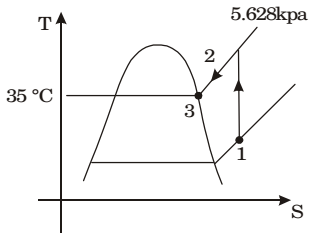## Refrigeration and Air-conditioning Miscellaneous

#### Refrigeration and Air-conditioning

1. A moist air sample has dry bulb temperature of 30°C and specific humidity of 11.5 g water vapour per kg dry air. Assume molecular weight of air as 28.93. If the saturation vapour pressure of water at 30°C is 4.24 kPa and the total pressure is 90 kPa, then the relative humidity (in%) of air sample is

1. Given: DBT = 30°C, w = 11.5 g/kg
∴ Relative humidity

 = Specific humidity × Total pressure 0.622 saturation vapour pressure

 φ = w × Pa 0.622 Pvs

 φ = 11.5 × 90 = 0.385 0.622 × 1000 × 4.24

φ ≈ 38.5%

##### Correct Option: B

Given: DBT = 30°C, w = 11.5 g/kg
∴ Relative humidity

 = Specific humidity × Total pressure 0.622 saturation vapour pressure

 φ = w × Pa 0.622 Pvs

 φ = 11.5 × 90 = 0.385 0.622 × 1000 × 4.24

φ ≈ 38.5%

1. Moist air at a pressure of 100 kPa is compressed to 500 kPa and then cooled to 35°C in an aftercooler. The air at the entry to the aftercooler is unsaturated and becomes just saturated at the exit of the aftercooler. The saturation pressure of water at 35°C is 5.628 kPa. The partial pressure of water vapour (in kPa) in the moist air entering the compressor is closest to

1. Assuming that compression is isentropic in air compressors, the process can be described on the T - s diagram. The process in the intercooler is constant pressure
p2 = p3
But p2 = 5p1p1 = p2 = p3 5 5

 p1 = 5.628 = 1.13kPa 5

##### Correct Option: B

Assuming that compression is isentropic in air compressors, the process can be described on the T - s diagram. The process in the intercooler is constant pressure
p2 = p3
But p2 = 5p1p1 = p2 = p3 5 5

 p1 = 5.628 = 1.13kPa 5

1. A building has to be maintained at 21 °C (dry bulb) and 14.5°C(wet bulb). The dew point temperature under these conditions is 10.17°C. The outside temperature is –23°C (dry bulb) and the internal and external surface heat transfer coefficients are 8 W/m2K and 23 W/ m2K respectively. If the building wall has a thermal conductivity of 1.2 W/mK, the minimum thickness (in m) of the wall required to prevent condensation is

1. NA

##### Correct Option: B

NA

1. For a typical sample of ambient air (at 30°C, 75% relative humidity and standard atmospheric pressure), the amount of moisture in kg per kg of dry air will be approximately.

1. Given data:
Dry bulb temperature:
Tdb = 35°C
Relative humidity
φ = 75° = 0.75
At 35°C, Ps = .05628 bar

 φ = Pv ⇒ 0.75 = Pv Ps .05628

Pv = 0.75× .05628 =.04221 bar
Specific humidity,
 ω = .622Pv = .622 × .04221 P - Pv 1.0135 - .04221

= 0.0270 kg/kg of dry air.

##### Correct Option: B

Given data:
Dry bulb temperature:
Tdb = 35°C
Relative humidity
φ = 75° = 0.75
At 35°C, Ps = .05628 bar

 φ = Pv ⇒ 0.75 = Pv Ps .05628

Pv = 0.75× .05628 =.04221 bar
Specific humidity,
 ω = .622Pv = .622 × .04221 P - Pv 1.0135 - .04221

= 0.0270 kg/kg of dry air.

1. Dew point temperature of air at one atmospheric pressure (1.013 bar) is 18°C. The air dry bulb temperature is 30°C. The saturation pressure of water at 18°C and 30°C are 0.02062 bar and 0.04241 bar respectively. The specific heat of air and water vapour respeetively are 1.005 and 1.88 kJ/kgK and the latent heat of vaporization water of 0°C is 2500 kJ/kg. The specific humidity (kg/kg of dry air) and enthalpy (kJ/kg of dry air) of this moist air respectively, are

1. Given: p = 1.013 bar, (pυ)tdp = 0.02062

 Specific humidity = 0.622 Pυ p - Pυ

 = 0.622 × 0.02062 = 0.01291 kg/kg of dry air 1.013 - 0.02062

h = 1.022 td + w(hfgdp + 2.3tdp)
= 63.15 kJ/kg of dry air.
Alternative method
pε = Saturation pressure = 0.02062 bar
pa = Partial pressure of air = 0.04241 bar
td = Dry bulb temperature = 30º
tw = Wet bulb temperature = 18º
Specific humidity,
 W = pυ × 0.622 = 0.01292 kg/kg of dry air. p - pυ

Total enthalpy,
h = cpa td + whs + wc ps (td – tω)
= 1.005 × 30 + 0.0129 × 2500 + 0.01292 × 1.88(30 – 18)
= 63.15 kJ/kg of dry air.

##### Correct Option: B

Given: p = 1.013 bar, (pυ)tdp = 0.02062

 Specific humidity = 0.622 Pυ p - Pυ

 = 0.622 × 0.02062 = 0.01291 kg/kg of dry air 1.013 - 0.02062

h = 1.022 td + w(hfgdp + 2.3tdp)
= 63.15 kJ/kg of dry air.
Alternative method
pε = Saturation pressure = 0.02062 bar
pa = Partial pressure of air = 0.04241 bar
td = Dry bulb temperature = 30º
tw = Wet bulb temperature = 18º
Specific humidity,
 W = pυ × 0.622 = 0.01292 kg/kg of dry air. p - pυ

Total enthalpy,
h = cpa td + whs + wc ps (td – tω)
= 1.005 × 30 + 0.0129 × 2500 + 0.01292 × 1.88(30 – 18)
= 63.15 kJ/kg of dry air.